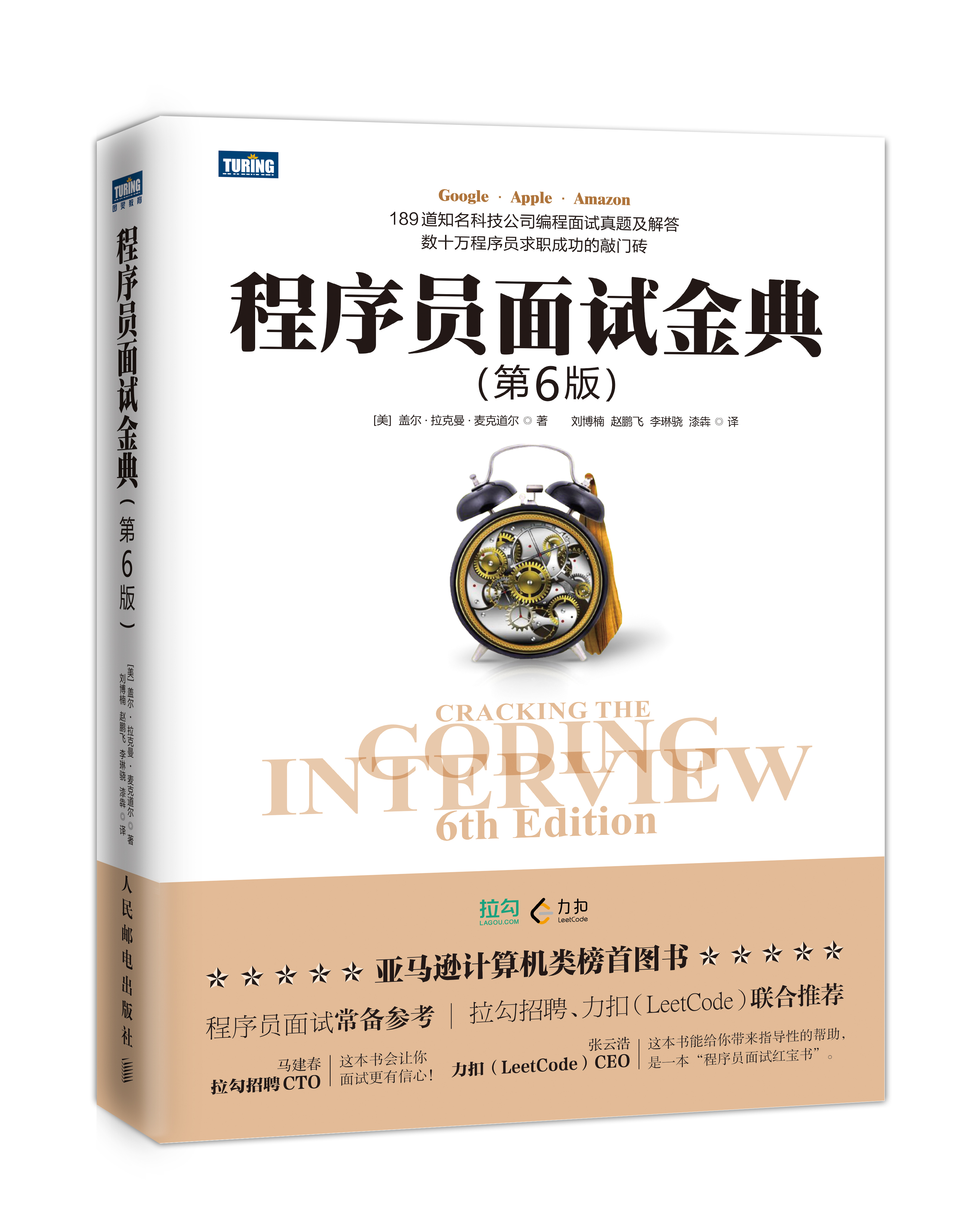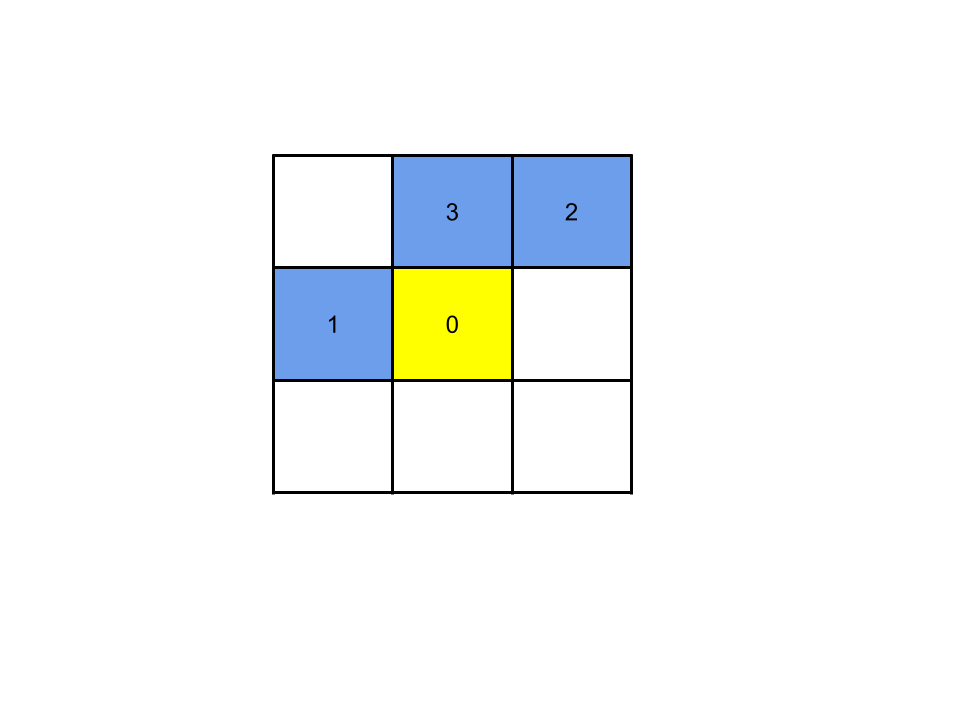🏆2019 力扣杯 - 全国秋季编程大赛### 1. 猜数字

class Solution:
def game(self, guess: List[int], answer: List[int]) -> int:
res = 0
for i in range(3):
res += 1
return res


### 2. 分式化简

class Solution:
def fraction(self, cont: List[int]) -> List[int]:
length = len(cont)
res = [cont[length - 1], 1]
for i in range(length - 2, -1, -1):
# 取反
cur = cont[i]
left = cur * res
res = [res + left, res]

return res


### 3. 机器人大冒险

1. 把执行一次命令能走到的点都记录下来，记为 path
2. 把命令中 R 的次数记作 pxU 的次数记作 py

r = min(x // px, y // py)


class Solution:
def robot(self, command: str, obstacles: List[List[int]], x: int, y: int) -> bool:
path = set()

px = 0
py = 0
for c in command:
if c == "R":
px += 1
else:
py += 1

def can_arrive(x, y):
x_round = x // px
y_round = y // py
r = min(x_round, y_round)
lx = x - r * px
ly = y - r * py
if (lx, ly) in path:
return True
else:
return False

if not can_arrive(x, y):
return False

for ob in obstacles:
if ob > x or ob > y:
continue
if can_arrive(ob, ob):
return False

return True


### 4. 覆盖

1. 放骨牌
1. 横着放
2. 竖着放
2. 不放骨牌
1. 不是坏格子，选择不放
2. 是坏格子，不可以放1. 如果格子 0 是一个坏掉的格子：不能放置骨牌
2. 如果格子 0 不是一个坏格子：
1. 如果上侧的格子 3 是一个未被占用的好格子：格子 0 可以竖放骨牌
2. 如果左侧的格子 1 是一个未被占用的好格子：格子 0 可以横放骨牌
3. 如果上侧格子 3 和左侧格子 1 都无法放置骨牌：格子 0 无法放置骨牌

#### 状态表示

• 0 表示格子不放置骨牌
• 1 表示格子放置骨牌

m 个格子就有 $2^m$ 种状态。

#### 状态转移

1. 坏格子：砍掉状态最高位 -> 左移 1 位 -> 最低位置 1
2. 不放骨牌：砍掉状态最高位 -> 左移 1 位
3. 横放骨牌：砍掉状态最高位 -> 左移 1 位 -> 最低两位置 1
4. 竖放骨牌：砍掉状态最高位 -> 左移 1 位 -> 最低位置 1

#### 步骤总结

1. 从左往右、从上往下遍历格子（意图为计算下一个状态 next_dp
2. 在当前格子中遍历所有 $2^m$ 种状态
3. 若状态非法，继续步骤 2
4. 若状态合法，根据状态转移计算出下一个状态 next_state 以及该状态下对应的 dp

#### 实现

class Solution:
def domino(self, n: int, m: int, broken: List[List[int]]) -> int:
# broken 记录
broken_map = dict()
for b in broken:
if b not in broken_map:
broken_map[b] = dict()
broken_map[b][b] = True

"""
是坏格子
"""
def is_broken(a, b):
if a in broken_map and b in broken_map[a]:
return True
else:
return False

"""
获取下一个状态
put: 0-不放，1-横放，2-竖放，3-坏格子
"""
def next_state(state, put):
# 去除高位
state = ~(1 << (m - 1)) & state

if put == 0:
# 不放牌
return state << 1
elif put == 1:
# 横着放
return ((state | 1) << 1) | 1
elif put == 2:
# 竖着放
return (state << 1) | 1
else:
# 坏格子
return (state << 1) | 1

# 创建 dp
dp = [-1] * (1 << m)
dp[(1 << m) - 1] = 0 # 全 1 的置 0

for i in range(n):
for j in range(m):
next_dp = [-1] * (1 << m)
# 遍历所有状态
for state in range(1 << m):
# 非法状态
if dp[state] == -1:
continue

if is_broken(i, j):
# 是坏格子
next_dp[next_state(state, 3)] = max(next_dp[next_state(state, 3)], dp[state]) # 是从 dp 转义还是保持现状
continue

# 不放
next_dp[next_state(state, 0)] = max(next_dp[next_state(state, 0)], dp[state])

# 可以竖放
if i != 0 and (1 << (m - 1)) & state == 0:
next_dp[next_state(state, 2)] = max(next_dp[next_state(state, 2)], dp[state] + 1)

# 可以横放
if j != 0 and 1 & state == 0:
next_dp[next_state(state, 1)] = max(next_dp[next_state(state, 1)], dp[state] + 1)

dp = next_dp

return max(dp)


### 5. 发 LeetCoin

1. 节点的父节点（用于向上更新父节点值）parent
2. 节点和其子节点的 coin 总数 coin
3. 通过操作 2 操作该节点时下发的 coin 数量 children_coin
4. 该节点包含的子节点数 count

#### 操作 1

1. 更新 node 节点的 coin += val
2. 更新其所有父节点的 coin += val

#### 操作 2

1. 更新 node 节点的 coin += all_coin
2. 更新 node 节点的所有父节点 coin += all_coin
3. 更新 node 节点的 children_coin += val

#### 操作 3

node 节点与其子节点的所有 LeetCoin 和为：

node.coin + (node.parent.children_coin * node.count + node.parent.parnet.children_coin * node.count ......)


#### 代码实现

class Node:
def __init__(self):
self.parent = None # 父节点
self.children = [] # 子节点数组
self.coin = 0 # 子树总coin
self.children_coin = 0 # 2 操作分配的 coin（影响到所有子树）
self.count = 1 # 子树节点数

class Solution:
def bonus(self, n: int, leadership: List[List[int]], operations: List[List[int]]) -> List[int]:
# 初始化数据
nodes = dict()
for i in range(1, n + 1):
nodes[i] = Node()

# 记录从属关系
wnode = nodes[worker]
lnode.children.append(wnode)
wnode.parent = lnode

"""
计算 count
"""
def cal_count(root):
for child in root.children:
cal_count(child)
root.count += child.count
return

"""
更新父项
"""
def update_parents(node, coin):
while node:
node.coin += coin
node = node.parent

"""
获取查询结果
"""
def get_res(node):
res = node.coin
node_count = node.count
parent = node.parent
while parent:
res += parent.children_coin * node_count
parent = parent.parent
return res

# 计算 count
cal_count(nodes)

res = []
for op in operations:
worker = op
if op == 1:
# 操作 1：个人发放
nodes[worker].coin += op
update_parents(nodes[worker].parent, op) # 更新它的所有父节点
elif op == 2:
# 操作 2：团队发放
all_coin = nodes[worker].count * op
# 当前节点加上数量
nodes[worker].children_coin += op
nodes[worker].coin += all_coin
# 更新父节点
update_parents(nodes[worker].parent, all_coin)
else:
res.append((get_res(nodes[worker])) % (10**9 + 7))
return res

10